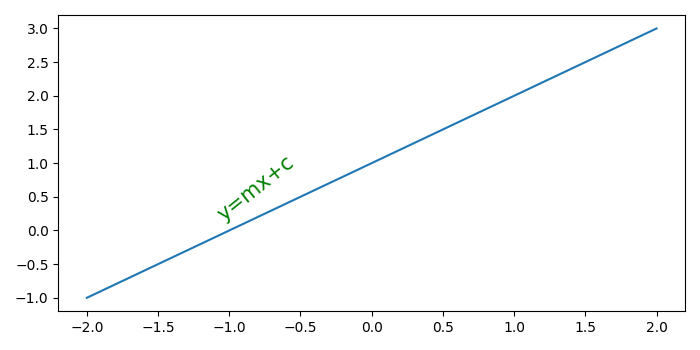# How to rotate Matplotlib annotation to match a line?

To rotate matplotlib annotation to match a line, we can take the following steps−

• Create a new figure or activate an existing figure using figure() method.
• Add an '~.axes.Axes' to the figure as part of a subplot arrangement using add_subplot() method.
• Initialize the variables, m (slope) and c (intercept).
• Create x and y data points using numpy.
• Calculate theta to make text rotation.
• Plot the line using plot() method with x and y.
• Place text on the line using text() method.
• To display the figure, use show() method.

## Example

import numpy as np
from matplotlib import pyplot as plt
plt.rcParams["figure.figsize"] = [7.00, 3.50]
plt.rcParams["figure.autolayout"] = True
fig = plt.figure()
m = 1
c = 1
x = np.linspace(-2, 2, 10)
y = m*x + c
theta = np.arctan(m)
line, = ax.plot(x, y)
ax.text(x=x, y=y+.25, s="y=mx+c", rotation=180.0*(1-theta), fontsize=15, color='green')
plt.show()

## Output